The correct solution to a geometrical probability problem often hinges on proper interpretation of the word "random." In Buffon's needle problem this was relatively straightforward; in other settings it is often not so easy.

J. Bertrand (1907) offers a good illustration of the potential pitfalls, plus allowing us to demonstrate our proficiency in deriving distributions of functions of random variables [BERT 07]. The problem is as follows:

Determine the probability that a "random chord" of a circle of unit radius has a length greater than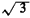, the side of an inscribed equilateral triangle (Figure 3.20).In urban applications, the "chord" may be a street, highway, pipeline, river, railroad, communication link, and so on. The circle may be the locus of points for which a helicopter may travel to in t minutes, or it may be the area of high pollution from a proposed plant, and so on. The threshold criterion that the chord exceedin length could refer to adequate coverage of a highway by a helicopter, the extent of a pollution effect from an industrial plant, and so on.

There are three plausible solutions to this problem.

Solution 1: Chord's end points are random. Any chord is uniquely determined by its end points intersecting the circle. These we may assume are uniformly independently distributed over the circumference of the circle. Without loss of generality, we may position ourselves at one of them and examine the relative location of the other. Imagining a vertex of an equilateral inscribed triangle at the conditioning point, the second point must fall on the one-third of the circumference linking the other two vertices. Thus, the probability is 1/3

Solution 2: Distance from the center is random. The length of any chord depends on its distance from the center of the circle and not on its direction. We may therefore assume that it has a fixed direction perpendicular to a given diameter of the circle and that its point of intersection with this diameter has a uniform distribution. For the chord to have a length greater thanthe distance of the point of intersection from the center of the circle must be less than 1/2, so that the probability is 1/2.

Solution 3: Point of intersection with a perpendicular is random over the circle. Any chord is uniquely defined by the location of the intersection of a perpendicular line extending from it to the circle center. We may suppose that the location of this intersection is uniformly distributed over the entire circle. Thus, the probability that this intersection is within a distance r of the center isr2 /(1)2 = r2 For the chord to have length greater thanthe intersection point must lie within a circle of radius r = 1/2 and thus the probability is 1/4.

All three solutions are "correct," but each refers to a different experiment. Recall that an experiment is characterized by a sample space and its accompanying probability assignment. The outcome of each experiment can be described as a value for, the angle of the chord with respect to some fixed coordinate system (0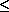2), and R, the distance from the circle center to the chord (0R1). Thus, the joint sample space, shown in Figure 3.21, corresponds to all points in the "rectangle" [002, 0r1].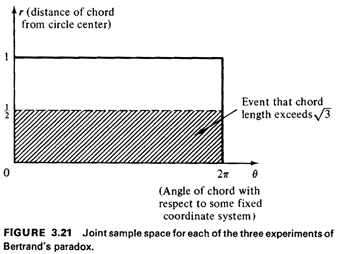Since the sample spaces (finest-grained list of outcomes) for all three experiments are identical, the probability assignments must differ due to different interpretations of the word "random." Let us reanalyze the three experiments to derive the accompanying probability assignment of each. We seek to find the joint probability density function for E) and R, f.,,,(O, r). For each of the three experiments we can argue that symmetry demands that E) be uniformly distributed between 0 and 2. Moreover, knowing the value of 0 tells us nothing about the value of R. Thus, 0 and R must be independent. The joint density function can then be expressed as a function of the individual marginal pdf's,Our task is thus reduced to finding the marginal pdf for R.

Solution 1: Chord's end points are random. Without loss of generality we can condition our analysis on a particular point of intersection for one end of the chord, letting the other end be randomly located (Figure 3.22).The relative angle between the two intersection points, measured from the circle center, is (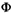= 2 cos-1 R. By the problem statement, (is uniformly distributed between 0 and. Again by symmetry, we can without loss of generality condition (to be in the interval [0,]. We now proceed in the usual stepwise (never-fail) fashion to find the pdf for R by first finding its cdf:Why was the direction of the inequality changed in the last step? Since 0 is uniformly distributed between 0 and,The probability that a "random" chord exceedsin length is equal to the integral of f,R (O, r) in the joint, R sample space corresponding to R < 1/2 (Figure 3.21). Because of the angular symmetry of the problem, we see that this probability is simply equal to the cdf of R evaluated at R = 1/2,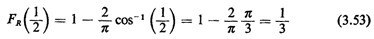as argued intuitively earlier.

Solution 2: Distance from the center is random.

In this case, by the problem statement, R is uniformly distributed between 0 and 1. Thus,By inspection the probability that a "random" chord exceedsin length is equal to 1/2.

Solution 3: Point of intersection with a perpendicular is random over the circle.
In this situation the "floating end" of the perpendicular whose length is R is uniformly distributed over the circle. Thus,Thus, we see that each solution was "correct," given its underlying probability assignment over the sample space. Geometrical problems that arise in an urban setting can have equally confounding alternative interpretations; thus, the urban analyst must take care to list his or her assumptions explicitly and to question problem statements employing the word "random."